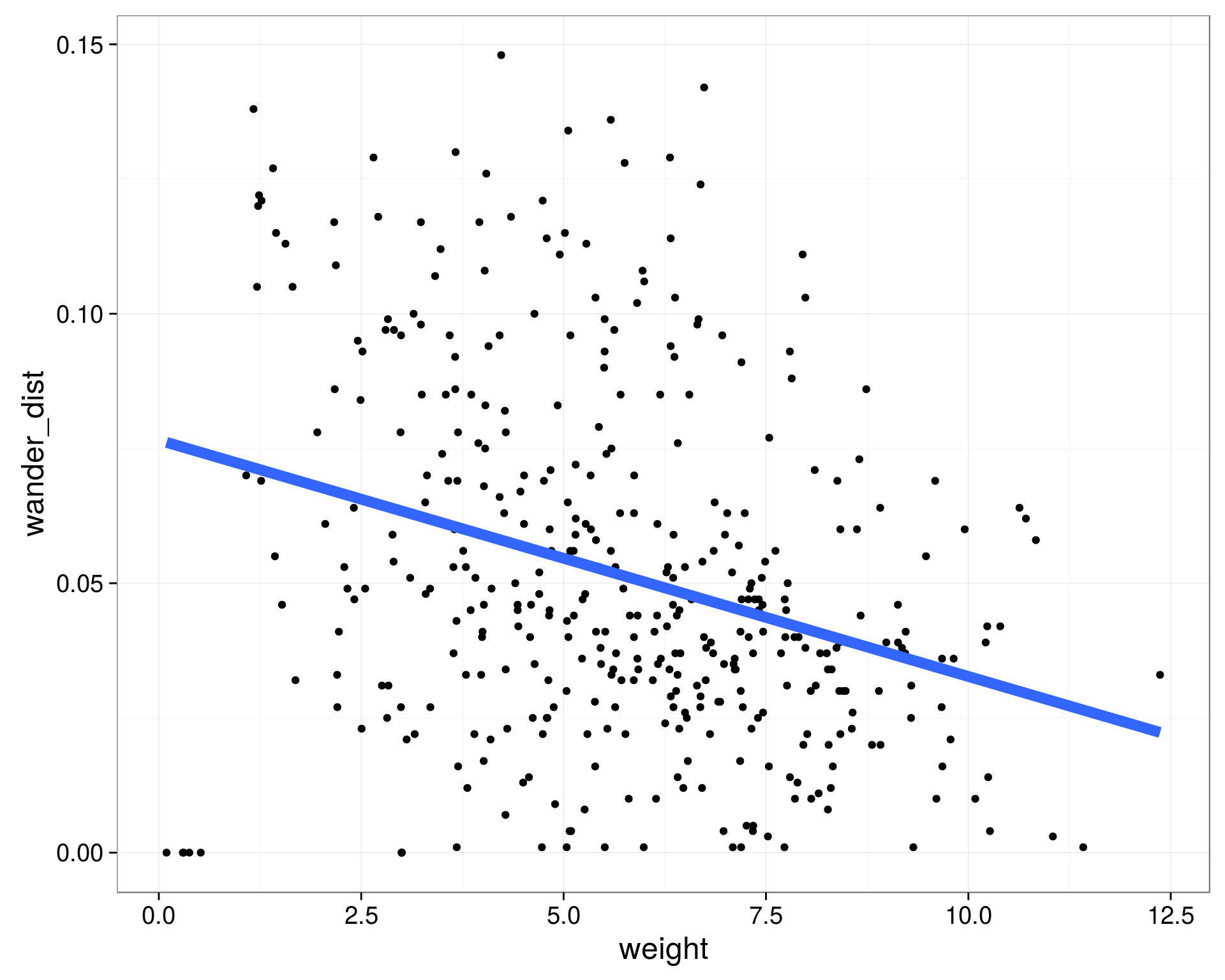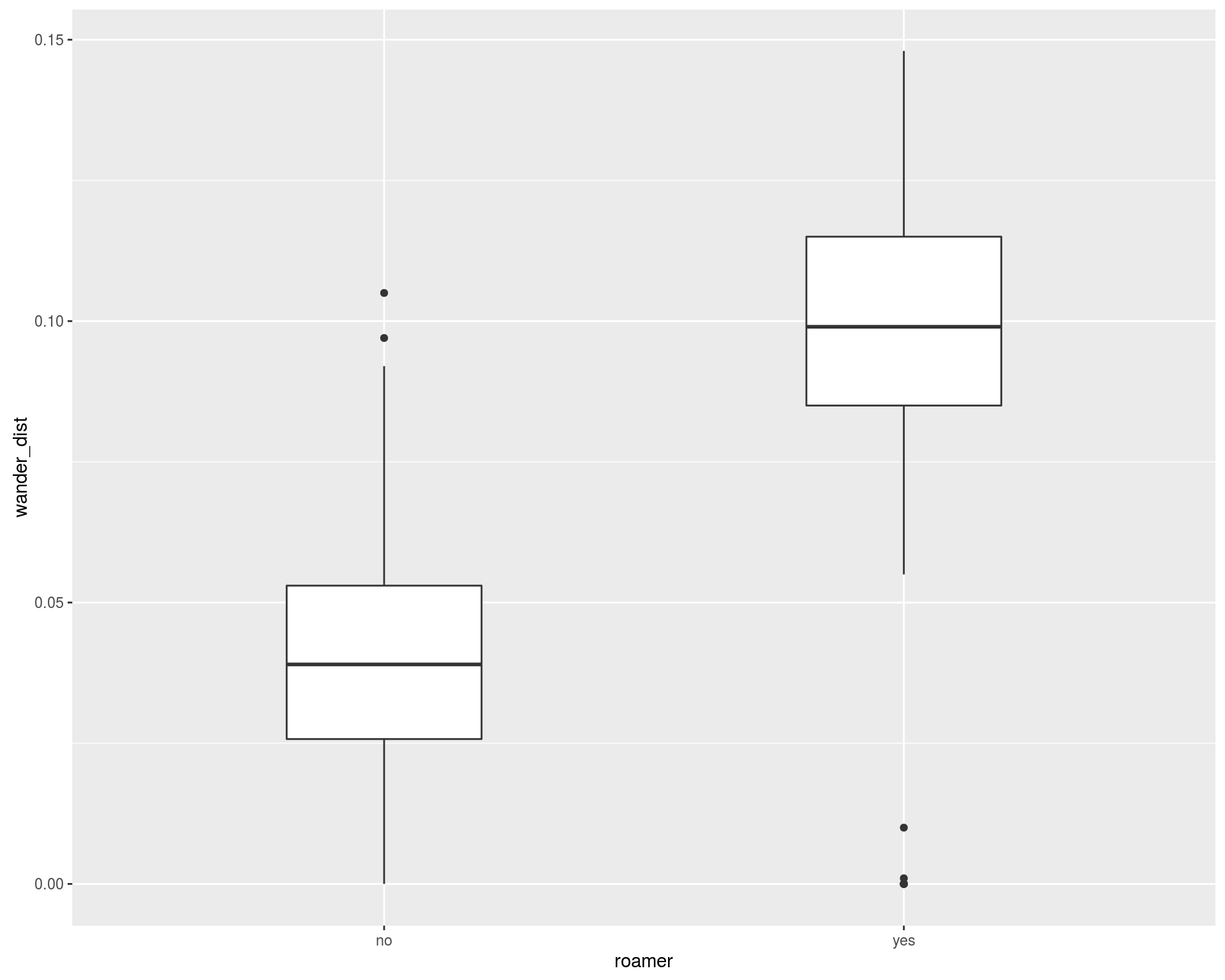## Learning Objectives

• To become familiar with common statistical functions available in R
• Linear Regression
• Logistic Regression
• K-Means Clustering

### Simple Linear RegressionLinear regression is one of the most commonly used methods in all of statistics. It is used for a large variety of applications and offers highly interpretable results. It was the first regression method discovered and belongs to one of the most important families of models, generalized linear models.

Simple linear regression estimates the linear relationship between two variables, an outcome variable y, and an explanatory variable x.

To fit a linear regression in R, we can use the `lm()` function (think linear model). We use the formula notation, `y~x` where `y` is the name of your outcome variable, and `x` is the name of your explanatory variable, both are unquoted. The easiest way to view the results interactively is with the `summary()` function.

``````weight_fit <- lm(formula = wander_dist ~ weight, data = cats)
summary(weight_fit)``````
``````
Call:
lm(formula = wander_dist ~ weight, data = cats)

Residuals:
Min        1Q    Median        3Q       Max
-0.076139 -0.019173 -0.004538  0.018240  0.094993

Coefficients:
Estimate Std. Error t value Pr(>|t|)
(Intercept)  0.0765553  0.0042990  17.808   <2e-16 ***
weight      -0.0043866  0.0006988  -6.278    9e-10 ***
---
Signif. codes:  0 '***' 0.001 '**' 0.01 '*' 0.05 '.' 0.1 ' ' 1

Residual standard error: 0.0316 on 398 degrees of freedom
Multiple R-squared:  0.09009,   Adjusted R-squared:  0.08781
F-statistic: 39.41 on 1 and 398 DF,  p-value: 9.003e-10``````

In this case, the `summary` function returns an object that provides a lot of interesting information when printed out. It also stores that information as part of the object, things like the terms used in the model, the coefficients of the model estimates, and the residuals of the model. This is nice if we want to do something programmatic with the results.

### Multiple Linear Regression

We aren’t restricted to just one explanatory variable in linear regression. We can test the effect of a linear relationship between multiple explanatory variables simultaneously. In the `lm` function, we just add extra variable names in the formula separated by `+`’s.

``````wander_fit <- lm(formula = wander_dist ~ weight + age , data = cats)
summary(wander_fit)``````
``````
Call:
lm(formula = wander_dist ~ weight + age, data = cats)

Residuals:
Min        1Q    Median        3Q       Max
-0.083498 -0.019287 -0.002461  0.017039  0.068823

Coefficients:
Estimate Std. Error t value Pr(>|t|)
(Intercept)  0.046857   0.004724   9.918   <2e-16 ***
weight      -0.014260   0.001119 -12.747   <2e-16 ***
age          0.017581   0.001661  10.587   <2e-16 ***
---
Signif. codes:  0 '***' 0.001 '**' 0.01 '*' 0.05 '.' 0.1 ' ' 1

Residual standard error: 0.02794 on 397 degrees of freedom
Multiple R-squared:  0.2904,    Adjusted R-squared:  0.2868
F-statistic: 81.24 on 2 and 397 DF,  p-value: < 2.2e-16``````

### Challenge

Fit a model predicting wander_dist and include weight, age, and fixed as predictors. What is the estimate for the effect of being fixed on the wandering distance?

``````wander_fit <- lm(formula = wander_dist ~ weight + age + fixed, data = cats)
summary(wander_fit)``````
``````
Call:
lm(formula = wander_dist ~ weight + age + fixed, data = cats)

Residuals:
Min        1Q    Median        3Q       Max
-0.091273 -0.018117 -0.001609  0.017690  0.071914

Coefficients:
Estimate Std. Error t value Pr(>|t|)
(Intercept)  0.054592   0.005074  10.760  < 2e-16 ***
weight      -0.014130   0.001101 -12.836  < 2e-16 ***
age          0.017582   0.001633  10.764  < 2e-16 ***
fixed       -0.011700   0.003083  -3.796  0.00017 ***
---
Signif. codes:  0 '***' 0.001 '**' 0.01 '*' 0.05 '.' 0.1 ' ' 1

Residual standard error: 0.02748 on 396 degrees of freedom
Multiple R-squared:  0.3153,    Adjusted R-squared:  0.3101
F-statistic: 60.79 on 3 and 396 DF,  p-value: < 2.2e-16``````

If an explanatory variable is not binary (coded as 0s or 1s), we can still include it in the model. The `lm` function understands factors to be categorical variables automatically and will output the estimates with a reference category.

``````wander_fit <- lm(formula = wander_dist ~ weight + age + factor(coat) + sex, data = cats)
summary_fit <- summary(wander_fit)
summary_fit['coefficients']``````
``````\$coefficients
Estimate  Std. Error     t value     Pr(>|t|)
(Intercept)          0.043847799 0.005622582   7.7985172 5.719688e-14
weight              -0.014196808 0.001125488 -12.6139100 7.470796e-31
age                  0.017760029 0.001675466  10.6000533 2.978738e-23
factor(coat)brown    0.006257836 0.004003785   1.5629800 1.188642e-01
factor(coat)calico  -0.001345917 0.005120491  -0.2628492 7.928049e-01
factor(coat)maltese  0.005543267 0.005907650   0.9383201 3.486577e-01
factor(coat)tabby    0.001579503 0.004265994   0.3702545 7.113928e-01
sexmale             -0.002616410 0.002870695  -0.9114206 3.626340e-01``````

### Challenge

What command will return the r-squared value from the summary_lm_fit object after running these commands:

wander_fit <- lm(formula = wander_dist ~ weight + age + fixed, data = cats) summary_lm_fit <- summary(wander_fit)

The `lm` function also can estimate interactions between explanatory variables. This is useful if we think that the linear relationship between our outcome y and a variable x1 is different depending on the variable x2. This can be accomplished by connecting two variables in the formula with a `*` instead of a `+`.

### Challenge

Fit a linear regression model estimating the relationship between the outcome, wandering distance (`wander_dist`) and explanatory variables age (`age`), weight (`weight`), with an interaction between age and weight. What is the coefficient associated with the interaction between age and weight?

``````wander_fit <- lm(formula = wander_dist ~ weight * age, data = cats)
summary(wander_fit)``````
``````
Call:
lm(formula = wander_dist ~ weight * age, data = cats)

Residuals:
Min        1Q    Median        3Q       Max
-0.070356 -0.019174 -0.002753  0.019351  0.066527

Coefficients:
Estimate Std. Error t value Pr(>|t|)
(Intercept)  0.0176044  0.0096232   1.829 0.068094 .
weight      -0.0086451  0.0019568  -4.418 1.29e-05 ***
age          0.0241292  0.0024971   9.663  < 2e-16 ***
weight:age  -0.0011310  0.0003255  -3.474 0.000569 ***
---
Signif. codes:  0 '***' 0.001 '**' 0.01 '*' 0.05 '.' 0.1 ' ' 1

Residual standard error: 0.02756 on 396 degrees of freedom
Multiple R-squared:  0.3114,    Adjusted R-squared:  0.3062
F-statistic: 59.69 on 3 and 396 DF,  p-value: < 2.2e-16``````

## Logistic Regression

If we are analyzing a binary outcome, we can use logistic regression. Logistic regression uses the linear model framework, but makes different assumptions about the distribution of the outcome. So we can look for associations between binary outcome variables and multiple explanatory variables.

``````ggplot(cats, aes(x = roamer, y = wander_dist)) +
geom_boxplot(width = 0.5)``````For logistic regression, we use the `glm` function. It takes formula and data arguments like the `lm` function, but we also need to specify a family. For logistic, we pass binomial as the family, which tells the `glm` function that we have a binary outcome, and we want to use the logit link function.

``roamer_fit <- glm(formula = roamer ~ wander_dist, data = cats, family = binomial )``

We can use the `summary` function to extract important information from the object that `glm` returns, just like with the `lm` function

``````glm_summary <- summary(roamer_fit)
glm_summary``````
``````
Call:
glm(formula = roamer ~ wander_dist, family = binomial, data = cats)

Deviance Residuals:
Min       1Q   Median       3Q      Max
-1.9068  -0.3812  -0.2234  -0.0767   3.4678

Coefficients:
Estimate Std. Error z value Pr(>|z|)
(Intercept)  -6.0103     0.5634 -10.668   <2e-16 ***
wander_dist  72.8672     7.4867   9.733   <2e-16 ***
---
Signif. codes:  0 '***' 0.001 '**' 0.01 '*' 0.05 '.' 0.1 ' ' 1

(Dispersion parameter for binomial family taken to be 1)

Null deviance: 421.53  on 399  degrees of freedom
Residual deviance: 212.89  on 398  degrees of freedom
AIC: 216.89

Number of Fisher Scoring iterations: 6``````

### Challenge

``````roamer_fit <- glm(formula = roamer ~ wander_dist + weight, data = cats, family = binomial )
summary(roamer_fit)``````
``````
Call:
glm(formula = roamer ~ wander_dist + weight, family = binomial,
data = cats)

Deviance Residuals:
Min       1Q   Median       3Q      Max
-2.2674  -0.3752  -0.1984  -0.0728   3.6661

Coefficients:
Estimate Std. Error z value Pr(>|z|)
(Intercept) -4.44909    0.75765  -5.872  4.3e-09 ***
wander_dist 68.99577    7.57569   9.108  < 2e-16 ***
weight      -0.25096    0.09484  -2.646  0.00814 **
---
Signif. codes:  0 '***' 0.001 '**' 0.01 '*' 0.05 '.' 0.1 ' ' 1

(Dispersion parameter for binomial family taken to be 1)

Null deviance: 421.53  on 399  degrees of freedom
Residual deviance: 205.44  on 397  degrees of freedom
AIC: 211.44

Number of Fisher Scoring iterations: 6``````

We can look at the effects of multiple covariates on our binary outcome with logistic regression, just like with linear regression. We just add as many variable names as we’d like to the right side of the formula argument, separated by `+` symbols.

``````ggplot(cats, aes(x = weight, y = wander_dist, color = roamer)) +
geom_point(size = 2) +
geom_smooth(method = 'lm', se = FALSE, size = 3) +
theme_bw(base_size = 18)``````